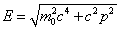# For what Z values does an atom begin to differ from a nonrelativistic model?

hb1547

## Homework Statement

"Relativistic effects are rather small in the hydrogen atom, but not so in higher-Z atoms. Estimate at what value of Z relativistic effects might alter energies by about a percent and whether it applies equally to all orbiting electrons or to some more than others. For this crude guess, it is acceptable to combine quantum mechanical results you have learned, related to energy, angular momentum, and/or probable radii, with some classical relationships."

My professor also added the note:
"You can consider the electrons to be orbiting in*a circular*orbit according to the Bohr model. Ignore screening in your calculation, but mention what effect it would have on a more realistic calculation."

## Homework Equations

$$E_{n} = -E_{0}\frac{Z^{2}}{n^{2}}$$

## The Attempt at a Solution

Just generally not sure where to begin with this. Do I look at it from a Bohr model standpoint, and try to find a point where Gamma becomes 1.01? Do I look at it from a quantum mechanical view, and try to use quantum numbers to find an energy level high enough that implies an electron is going a certain speed?

soothsayer
I would do the latter, the equation you posted is a good one, you have to be able to relate the Energy of the orbiting electron with its velocity; you can plug in gamma = 1.01 the relativistic momentum equation and use:to find the corresponding energy, then plug that into E in the equation you posted and Z=1 for hydrogen and solve for n!

Also, do you go to UC Santa Cruz? It sounds like you're in the same class as me... ;)

#### Attachments

hb1547
Gotta love Professor Smith

soothsayer
Yeah, he's pretty awesome.

(Also, that equation I gave is for total E, you need KE and it's actually way easier to use KE = (gamma)mc2-mc2, you don't need to calculate momentum or velocity)

hb1547
Awesome thanks! That helped a lot -- that was a much simpler problem than I was making it in my head. Thanks again, good luck on tomorrows quiz, haha